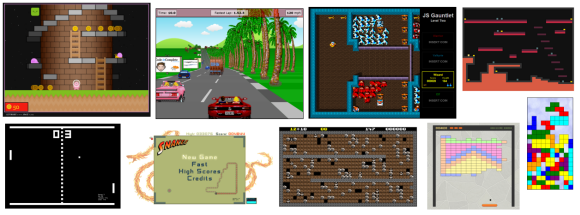# Javascript Game Foundations - Math

Fri, Dec 6, 2013## Math

Sorry, but it’s inevitable that all game programmers will eventually need to do math. Just as night follows day, sure as eggs is eggs, just like every odd-numbered Star Trek movie is s**t (ref)

The kind of math we will need is going to depend entirely on the type of game we are making. Are there moving objects? accelerating objects? falling objects? Do we need collision detection? Are we making a 3d game?

We already have some simple helper methods in the standard `Math` object such as `min()`, `max()`, `abs()`, etc. Since it’s best practice to avoid extending built in objects we will probably want to provide our own namespaced helper module.

How about starting with some random number helper methods:

``````Game.Math = {

random: function(min, max) {
return (min + (Math.random() * (max - min)));
},

randomInt: function(min, max) {
return Math.round(this.random(min, max));
},

randomChoice: function(choices) {
return choices[this.randomInt(0, choices.length-1)];
},

randomBool: function() {
return this.randomChoice([true, false]);
},

...
}
``````

Now, depending on the requirements for our game, we might want to add other helper methods. The rest of this article presents just a few examples of the kind of math you’ll likely need…

## Simple Math

``````limit: function(x, min, max) {
return Math.max(min, Math.min(max, x));
},

between: function(n, min, max) {
return ((n >= min) && (n <= max));
},

accelerate: function(v, accel, dt) {
return v + (accel * dt);
},

lerp: function(n, dn, dt) {   // linear interpolation
return n + (dn * dt);
},
``````

## Easing Equations

We quite commonly need to animate a value. This is generally called easing (or sometimes, slightly incorrectly, called tweening). There are a number of different easing equations, the most common being easeIn and easeOut:

``````interpolate: function(a,b,percent) { return a + (b-a)*percent                                 },
easeIn:      function(a,b,percent) { return a + (b-a)*Math.pow(percent,2);                    },
easeOut:     function(a,b,percent) { return a + (b-a)*(1-Math.pow(1-percent,2));              },
easeInOut:   function(a,b,percent) { return a + (b-a)*((-Math.cos(percent*Math.PI)/2) + 0.5); },

// ... and many more
``````

## Color Manipulation

If we want dynamic lighting, then we will need a way to brighten, or darken, a color:

``````brighten: function(hex, percent) {

var a = Math.round(255 * percent/100),
r = a + parseInt(hex.substr(1, 2), 16),
g = a + parseInt(hex.substr(3, 2), 16),
b = a + parseInt(hex.substr(5, 2), 16);

r = r<255?(r<1?0:r):255;
g = g<255?(g<1?0:g):255;
b = b<255?(b<1?0:b):255;

return '#' + (0x1000000 + (r * 0x10000) + (g * 0x100) + b).toString(16).slice(1);
},

darken: function(hex, percent) {
return this.brighten(hex, -percent);
},
``````

## Collision Detection

Collision detection is a subject for a whole other series of articles, suffice to say you will need some math! Here are a couple of likely candidates:

``````overlap: function(box1, box2) {
return !((box1.right  < box2.left)   ||
(box1.left   > box2.right)  ||
(box1.top    > box2.bottom) ||
(box1.bottom < box2.top));
},

lineIntercept: function(x1, y1, x2, y2, x3, y3, x4, y4, d) {
var denom = ((y4-y3) * (x2-x1)) - ((x4-x3) * (y2-y1));
if (denom != 0) {
var ua = (((x4-x3) * (y1-y3)) - ((y4-y3) * (x1-x3))) / denom;
if ((ua >= 0) && (ua <= 1)) {
var ub = (((x2-x1) * (y1-y3)) - ((y2-y1) * (x1-x3))) / denom;
if ((ub >= 0) && (ub <= 1)) {
var x = x1 + (ua * (x2-x1));
var y = y1 + (ua * (y2-y1));
return { x: x, y: y, d: d };
}
}
}
return null;
},
``````

I’ve written in more detail about collision detection in a variety of javascript games:

And there are many more resources available on the web:

## 3d Graphics

Even if you just want to do some simple pseudo-3d effects, you will likely need some form of projection from 3-d space to 2-d space:

``````project: function(p, cameraX, cameraY, cameraZ, cameraDepth, width, height) {
p.camera.x     = (p.world.x || 0) - cameraX;
p.camera.y     = (p.world.y || 0) - cameraY;
p.camera.z     = (p.world.z || 0) - cameraZ;
p.screen.x     = Math.round((width/2)  + (p.screen.scale * p.camera.x  * width/2));
p.screen.y     = Math.round((height/2) - (p.screen.scale * p.camera.y  * height/2));
},
``````

You’ll probably want a Vector and Matrix class as well, but at that point I think you’re moving beyond a build-it-yourself project and probably should be researching frameworks and libraries such as three.js

There are a multitude of references, articles, books, and entire college degrees to cover the topic of 3-dimensional graphics - google

## More Math

The more games you develop, the more math you’ll need. Here are a few recommended books:

Good job you studied well at school huh ?

## Math vs Maths

I really, really wanted to add an s at the end of the word maths. I am english after all, but I’m also a U.S. citizen, so I’m conflicted. I guess this article could very well be the definining moment where I finally picked-a-side!